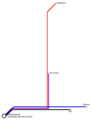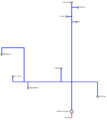# File:Seidenstrasse34c3.png

Original file(496 × 647 pixels, file size: 10 KB, MIME type: image/png)

Generation code for https://beno.org.uk/metromapcreator/

setroutes(2);curvenum = 2;jumpnum = 2.4;cowpatuuuuu = 0;fontzsize = 8;line1col = "#ff0000";line1width = 4;line1ver = 1;line1ver1x = 523;line1ver1y1 = 845;line1ver1y2 = 894;line1hor = 0;line1topleft = 0;line1topright = 0;line1stations = 1;line1station1x = 523;line1station1y = 894;line1station1type = 4;line1station1dir = 4;line1station1text = "vaccuum";line2col = "#0008ff";line2width = 4;line2ver = 7;line2ver1x = 523;line2ver1y1 = 54;line2ver1y2 = 849;line2ver2x = 712;line2ver2y1 = 632;line2ver2y2 = 740;line2ver3x = 446;line2ver3y1 = 534;line2ver3y2 = 632;line2ver4x = 93;line2ver4y1 = 593;line2ver4y2 = 632;line2ver5x = 176;line2ver5y1 = 383;line2ver5y2 = 632;line2ver6x = 12;line2ver6y1 = 383;line2ver6y2 = 432;line2ver7x = 204;line2ver7y1 = 632;line2ver7y2 = 677;line2hor = 8;line2hor1x1 = 523;line2hor1x2 = 568;line2hor1y = 89;line2hor2x1 = 485;line2hor2x2 = 523;line2hor2y = 156;line2hor3x1 = 523;line2hor3x2 = 558;line2hor3y = 194;line2hor4x1 = 523;line2hor4x2 = 712;line2hor4y = 632;line2hor5x1 = 446;line2hor5x2 = 523;line2hor5y = 632;line2hor6x1 = 390;line2hor6x2 = 446;line2hor6y = 632;line2hor7x1 = 93;line2hor7x2 = 401;line2hor7y = 632;line2hor8x1 = 12;line2hor8x2 = 176;line2hor8y = 383;line2topleft = 0;line2topright = 0;line2stations = 12;line2station1x = 523;line2station1y = 849;line2station1type = 5;line2station1dir = 4;line2station1text = "START (Art & Play)";line2station2x = 523;line2station2y = 54;line2station2type = 3;line2station2dir = 4;line2station2text = "Chaos West";line2station3x = 568;line2station3y = 89;line2station3type = 0;line2station3dir = 4;line2station3text = "unknown";line2station4x = 568;line2station4y = 89;line2station4type = 3;line2station4dir = 5;line2station4text = "unknown";line2station5x = 485;line2station5y = 156;line2station5type = 3;line2station5dir = 4;line2station5text = "Freifunk";line2station6x = 558;line2station6y = 194;line2station6type = 0;line2station6dir = 4;line2station6text = "Bar";line2station7x = 558;line2station7y = 194;line2station7type = 3;line2station7dir = 5;line2station7text = "Bar";line2station8x = 712;line2station8y = 740;line2station8type = 3;line2station8dir = 5;line2station8text = "Art & Play";line2station9x = 446;line2station9y = 534;line2station9type = 3;line2station9dir = 4;line2station9text = "C-Base";line2station10x = 12;line2station10y = 432;line2station10type = 3;line2station10dir = 5;line2station10text = "Kid Space 2";line2station11x = 93;line2station11y = 593;line2station11type = 3;line2station11dir = 5;line2station11text = "Kid Space 1";line2station12x = 204;line2station12y = 677;line2station12type = 3;line2station12dir = 5;line2station12text = "Jugend Hackt"

## File history

Click on a date/time to view the file as it appeared at that time.

Date/TimeThumbnailDimensionsUserComment
current22:52, 27 December 2017496 × 647 (10 KB)Alsternerd (talk | contribs)setroutes(4);curvenum = 2;jumpnum = 2.4;cowpatuuuuu = 0;fontzsize = 8;line1col = "#ff0000";line1width = 4;line1ver = 2;line1ver1x = -10;line1ver1y1 = -10;line1ver1y2 = -10;line1ver2x = 314;line1ver2y1 = 259;line1ver2y2 = 779;line1hor = 1;line1hor1x1 =...
17:04, 22 November 20171,827 × 2,048 (83 KB)Alsternerd (talk | contribs)Smaller dimensions to get the wiki to create a thumbnail. (Needs more ressources!)
17:02, 22 November 2017
Error creating thumbnail: File with dimensions greater than 12.5 MP
3,904 × 4,376 (154 KB)Alsternerd (talk | contribs)Generation code for https://beno.org.uk/metromapcreator/ setroutes(2);curvenum = 2;jumpnum = 2.4;cowpatuuuuu = 0;fontzsize = 8;line1col = "#ff0000";line1width = 4;line1ver = 1;line1ver1x = 523;line1ver1y1 = 845;line1ver1y2 = 894;line1hor = 0;line1topl...
• You cannot overwrite this file.

The following page links to this file: